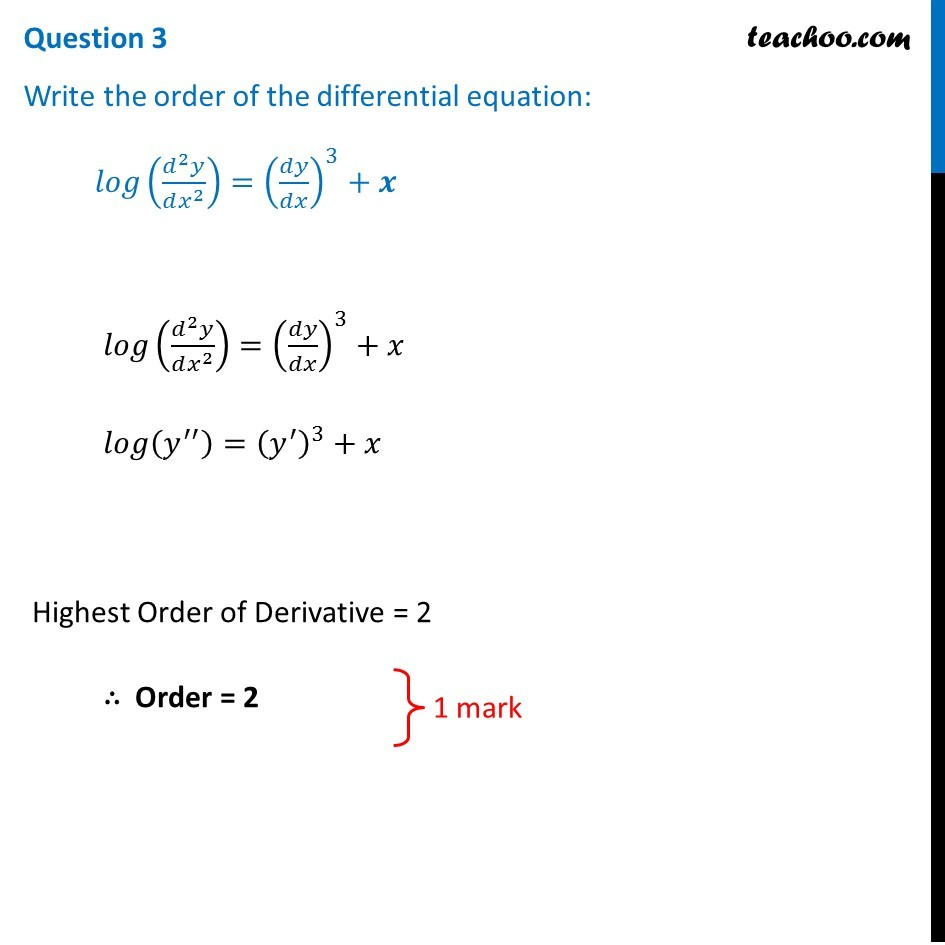CBSE Class 12 Sample Paper for 2019 Boards

Class 12
Solutions of Sample Papers and Past Year Papers - for Class 12 Boards

Question 3

Write the order of the differential equation:

log⁡ (d 2 y/dx 2 ) = (dy/dx) 3 + x### Transcript

Question 3 Write the order of the differential equation: 𝑙𝑜𝑔⁡((𝑑^2 𝑦)/(𝑑𝑥^2 ))=(𝑑𝑦/𝑑𝑥)^3+𝒙 𝑙𝑜𝑔⁡((𝑑^2 𝑦)/(𝑑𝑥^2 ))=(𝑑𝑦/𝑑𝑥)^3+ 𝑥 𝑙𝑜𝑔⁡(𝑦^′′ )=(𝑦^′ )^3+ 𝑥 Highest Order of Derivative = 2 ∴ Order = 2